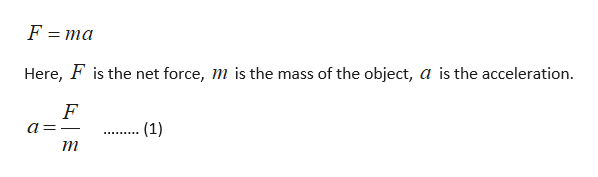# If the mass of sliding block is tripled while a constant net force is applied, by how much does the acceleration decrease?

Question
1 views

If the mass of sliding block is tripled while a constant net force is applied, by how much does the acceleration decrease?

check_circle

Step 1

The formula for the net f...help_outlineImage TranscriptioncloseF = ma Here, F is the net force, m is the mass of the object, a is the acceleration. - (1) ......... т fullscreen

### Want to see the full answer?

See Solution

#### Want to see this answer and more?

Solutions are written by subject experts who are available 24/7. Questions are typically answered within 1 hour.*

See Solution
*Response times may vary by subject and question.
Tagged in

### Physics